International
Tables for
Crystallography
Volume B
Reciprocal space
Edited by U. Shmueli

International Tables for Crystallography (2006). Vol. B. ch. 2.5, pp. 316-317   | 1 | 2 |

## Section 2.5.6.2. Orthoaxial projection

B. K. Vainshteinc

#### 2.5.6.2. Orthoaxial projection

| top | pdf |

In practice, an important case is where all the projection directions are orthogonal to a certain straight line: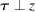(Fig. 2.5.6.3). Here the axis of rotation or the axis of symmetry of an object is perpendicular to an electron beam. Then the three-dimensional problem is reduced to the two-dimensional one, since each cross section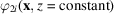is represented by its one-dimensional projections. The direction of vector τ is defined by the rotational angle ψ of a specimen: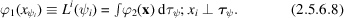In this case, the reconstruction is carried out separately for each level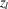: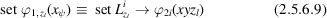and the three-dimensional structure is obtained by superposition of layers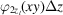(Vainshtein et al., 1968; Vainshtein, 1978).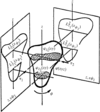Figure 2.5.6.3 | top | pdf |Orthoaxial projection.

### ReferencesVainshtein, B. K. (1978). Electron microscopical analysis of the three-dimensional structure of biological macromolecules. In Advances in optical and electron microscopy, Vol. 7, edited by V. E. Cosslett & R. Barer, pp. 281–377. London: Academic Press.Google ScholarVainshtein, B. K., Barynin, V. V. & Gurskaya, G. V. (1968). The hexagonal crystalline structure of catalase and its molecular structure. Sov. Phys. Dokl. 13, 838–841.Google Scholar